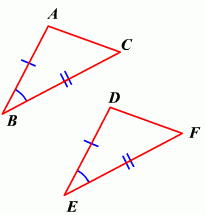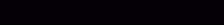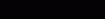# If You Are Going To Use The Sas Postulate To Prove These Two Triangles Congruent, What Additional Information Do You Need?

You need to know that two sides of the triangles and the angle that those two sides make are congruent.
thanked the writer.
First you need to understand that congruent means equal.

Next you need to know at least two side measures of each of the triangles you are comparing and you need to know the measure of the angle made by these two sides. If all of that information is the same on both triangles then you have two equal (congruent) triangles.

Below is a visual -  the tick marks indicate which sides are equal and the funny looking equal sign means congruent.In the figure,.
Therefore, by the SAS postulate,.
thanked the writer.